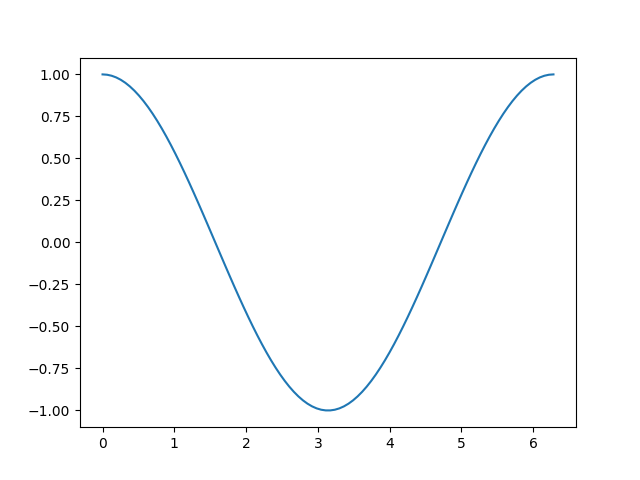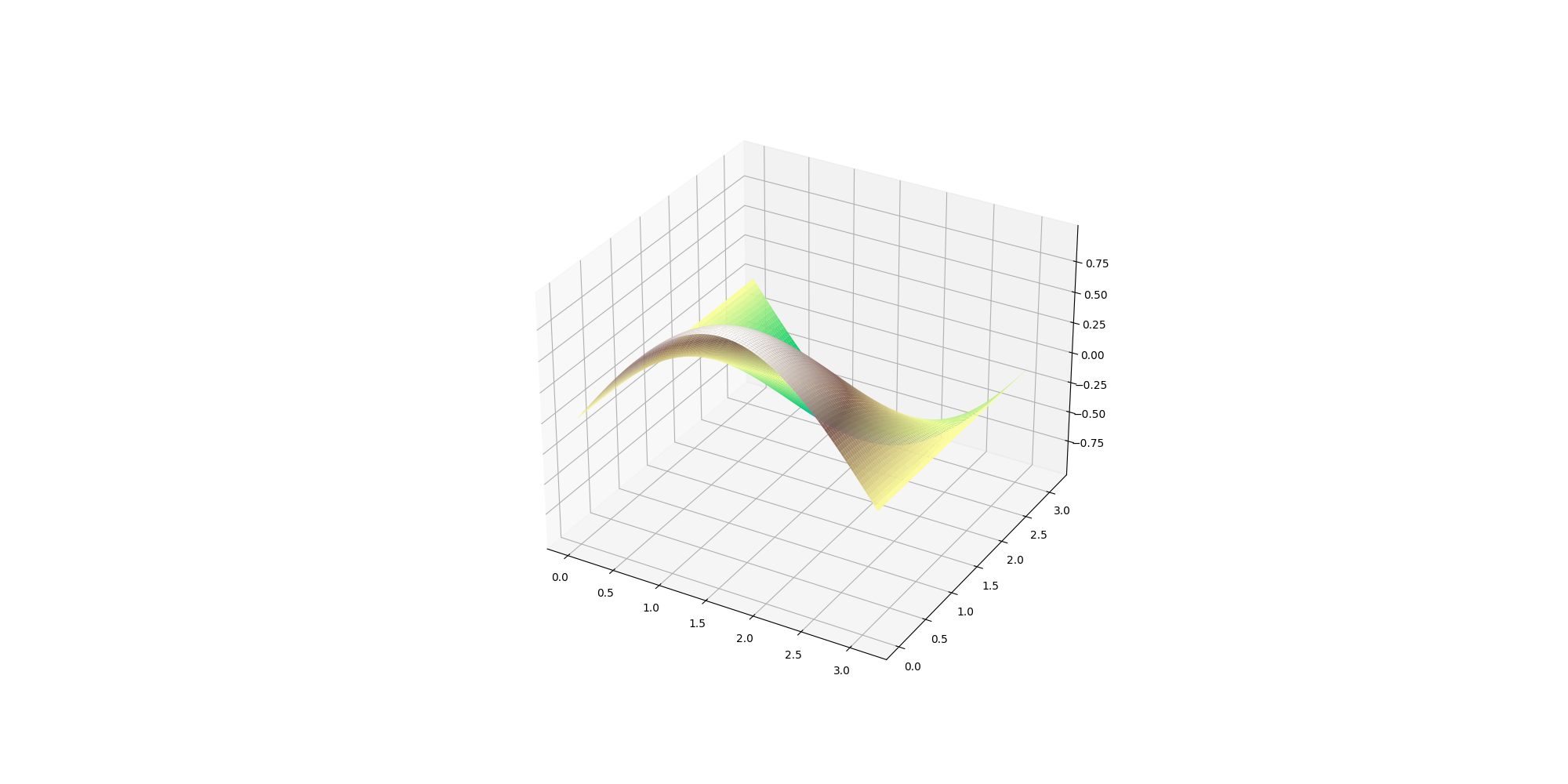a field-theory motivated approach to computer algebra

# Numerical evaluation of expressions

Cadabra is primarily a symbolic computer algebra system, in the sense that it focuses on symbolic expressions, not the numerical value they take when all symbols in them are replaced with values. However, Cadabra does have functionality to evaluate expressions numerically as well, using either a call through SymPy, or using its own internal expression evaluator. We will here focus on the latter, as it is by far the fastest. Let us start with a simple example to understand the basics. The following code creates a Cadabra expression containing just $\cos(x)$, and then numerically evaluates that expression for 100 values of $x$ in the range $[0, 2\pi]$.
import numpy as np import matplotlib.pyplot as plt
ex := \cos(x); xv = np.linspace(0, np.pi*2, 100) exv = nevaluate(ex, {$x$: xv} );
$$\displaystyle{}\cos{x}$$
\cos(x)
<cadabra2.NTensor object at 0xffffaf7f11b0>
The nevaluate function returns an NTensor, which is Cadabra's object to store numerical values of tensors. It can be converted to a numpy array, after which you can plot it:
plt.plot( xv, np.array(exv) );{}$\big[$$\big]$
To understand nevaluate, take a close look at the arguments. The first argument is the expression we want to evaluate. The second argument is a dictionary, in wich we list the symbols appearing in the expression (here just $x$) and the values that each such symbol takes (here it's the values in the array xv).

## More complicated examples

The example above evaluated a function of a single variable over a range of values of that variable. We can also use this to evaluate functions of multiple variables. The example below shows this.
ex:= \cos(x) \sin(y); xv = np.linspace(0, np.pi, 100) yv = np.linspace(0, np.pi, 100) z = np.array( nevaluate(ex, {$x$: xv, $y$: yv} ) )
$$\displaystyle{}\cos{x} \sin{y}$$
\cos(x) \sin(y)
The z variable is now a two-dimensional array, the first axis of which is the $x$-axis and the second the $y$-axis (more on this order below). We can plot such a data set by creating a meshgrid from the $x$ and $y$ values, and then feeding the lot into plot_surface:
XV, YV=np.meshgrid(xv, yv) plt.figure(figsize=(20, 10)) ax = plt.axes(projection='3d') plt.figure().subplots_adjust(top=1, bottom=0, left=0, right=1, wspace=0) ax.plot_surface(XV, YV, z, rstride=1, cstride=1, cmap='terrain', edgecolor=None);The order in which the axis of the result of nevaluate should be interpreted is determined by the order in which you list them in the values dictionary. Compare the following:
z1 = np.array( nevaluate(ex, {$x$: xv, $y$: yv} )) z2 = np.array( nevaluate(ex, {$y$: yv, $x$: xv} ))
z1[10,20]; z2[10,20];
0.5633046988744114
0.2512712531759243

## Supported elementary functions

At present the nevaluate function supports expressions with the following building blocks: multiplication/division, addition/subtraction, trigonometric functions, hyperbolic trigonometric functions, logarithms, exponential, square root.